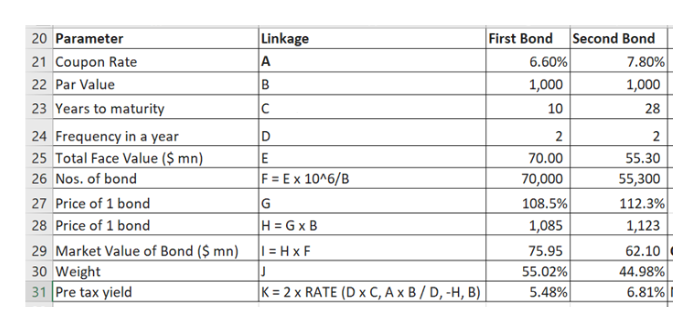# Hero Manufacturing has 8.5 million shares of common stock outstanding. The current share price is \$77 and the book value per share is \$6. The company also has two bond issues outstanding. The first bond issue has a face value of \$70 million, a coupon rate of 6.6 percent and sells for 108.5 percent of par. The second issue has a face value of \$55.3 million, a coupon rate of 7.8 percent and sells for 112.3 percent of par. The first issue matures in 10 years, the second in 28 years. Suppose the company’s stock has a beta of 1.4. The risk-free rate is 2.8 percent and the market risk premium is 6.9 percent. Assume that the overall cost of debt is the weighted average implied by the two outstanding debt issues. Both bonds make semiannual payments. The tax rate is 22 percent. What is the company’s WACC?

Question
608 views
 Hero Manufacturing has 8.5 million shares of common stock outstanding. The current share price is \$77 and the book value per share is \$6. The company also has two bond issues outstanding. The first bond issue has a face value of \$70 million, a coupon rate of 6.6 percent and sells for 108.5 percent of par. The second issue has a face value of \$55.3 million, a coupon rate of 7.8 percent and sells for 112.3 percent of par. The first issue matures in 10 years, the second in 28 years. Suppose the company’s stock has a beta of 1.4. The risk-free rate is 2.8 percent and the market risk premium is 6.9 percent. Assume that the overall cost of debt is the weighted average implied by the two outstanding debt issues. Both bonds make semiannual payments. The tax rate is 22 percent. What is the company’s WACC?
check_circle

star
star
star
star
star
1 Rating
Step 1

As a first step we need to calculate the weigthed average pre tax cost of debt based on the characterisitics of the two types of bonds the firm has. We then will calculate the cost of equity, proportion of debt and equity in the capital structure based on market value. We will then proceed to calculate the WACC.

Step 2

Please see the table on the white board. Please be guided by the second column titled “Linkage” to understand the mathematics. The calculations are sequential. I have used RATE function of excel to obtain the pre tax yield.

Weight of the first bond has been calculated as = 75.95 / (75.95 + 62.10) = 55.02% (You should be able to locate these figures in the snapshot on the white board)help_outlineImage TranscriptioncloseLinkage First Bond |Second Bond 6.60% 20 Parameter 7.80% 21 Coupon Rate A 22 Par Value 1,000 1,000 23 Years to maturity 24 Frequency in a year 25 Total Face Value (\$ mn) C 10 28 2 2 E 70.00 55.30 F = Ex 10^6/B 26 Nos. of bond 70,000 55,300 27 Price of 1 bond 108.5% 1,085 112.3% G H GxB 28 Price of 1 bond 1,123 29 Market Value of Bond (\$ mn) 30 Weight 31 Pre tax yield = H x F 75.95 62.10 55.02% 5.48% J 44.98% K = 2 x RATE (D x C, A x B / D, -H, B) 6.81% fullscreen
Step 3

Weighted average pre tax cost of debt, Kd = Weight of first bond x Pre tax yield of first bond + Weight of second bond x Pre tax yield of second bond = 55.02% x 5.48%...

### Want to see the full answer?

See Solution

#### Want to see this answer and more?

Solutions are written by subject experts who are available 24/7. Questions are typically answered within 1 hour.*

See Solution
*Response times may vary by subject and question.
Tagged in